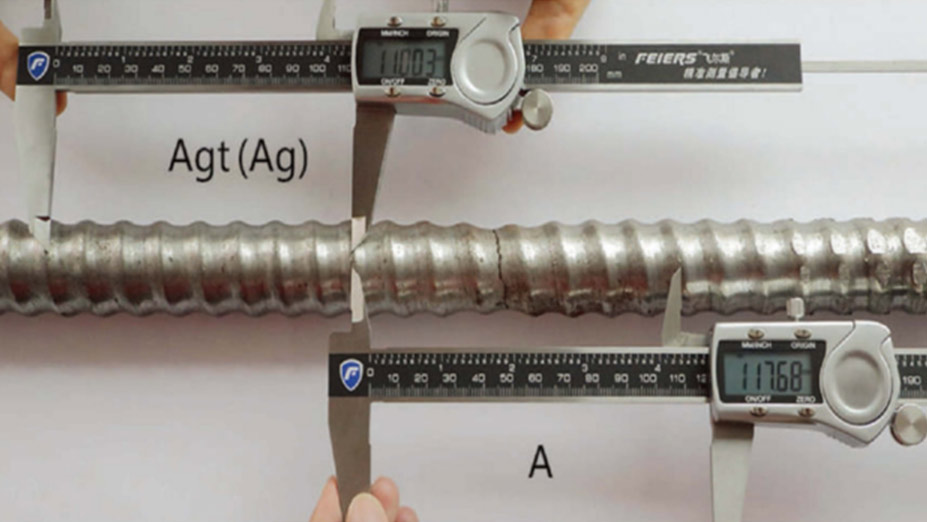#### How to measure AGT?

For the example shown in the picture above, Assume the tensile strength of this hollow bar, Rm = 800 N/mm2 Measurement (as shown) and calculated:

Ag= {(110.03mm-100mm) I 100mm}*100 = 10 Rm/ 2000 = 800/ 2000 = 0.4
Agt = Ag+ Rm/ 2000 = 10.4
A = {(117.68mm-100mm) I 100mm}*100 = 17.6
Result: The elongation of the hollow bar: Agt = 10.4{%) A = 17.6(%)

（By Manual Method）